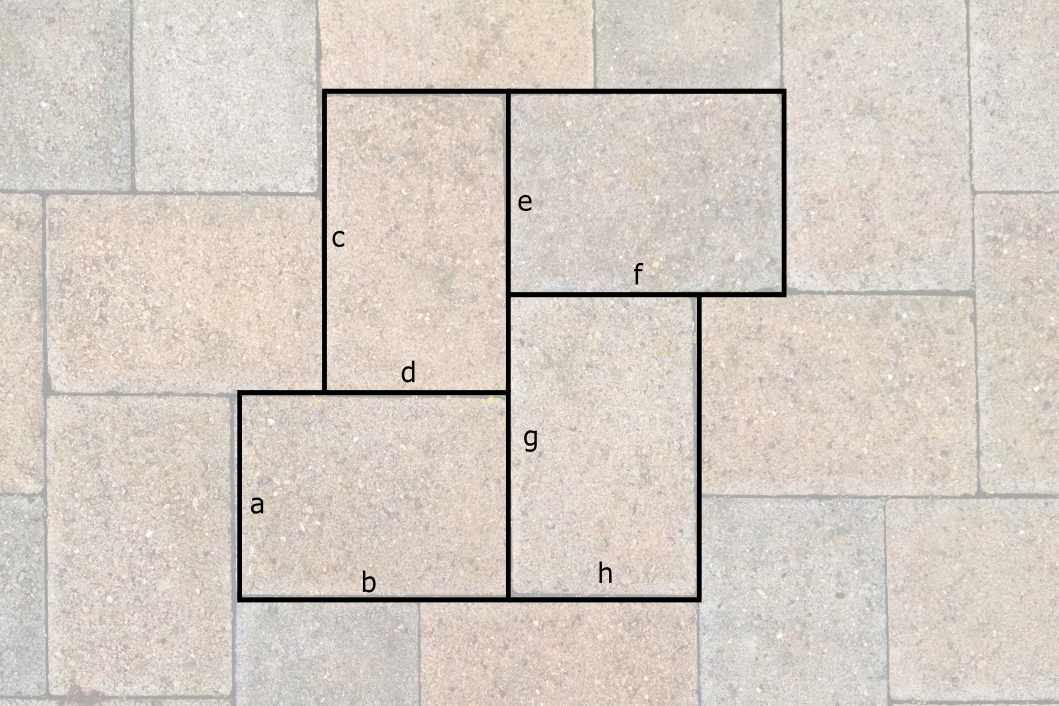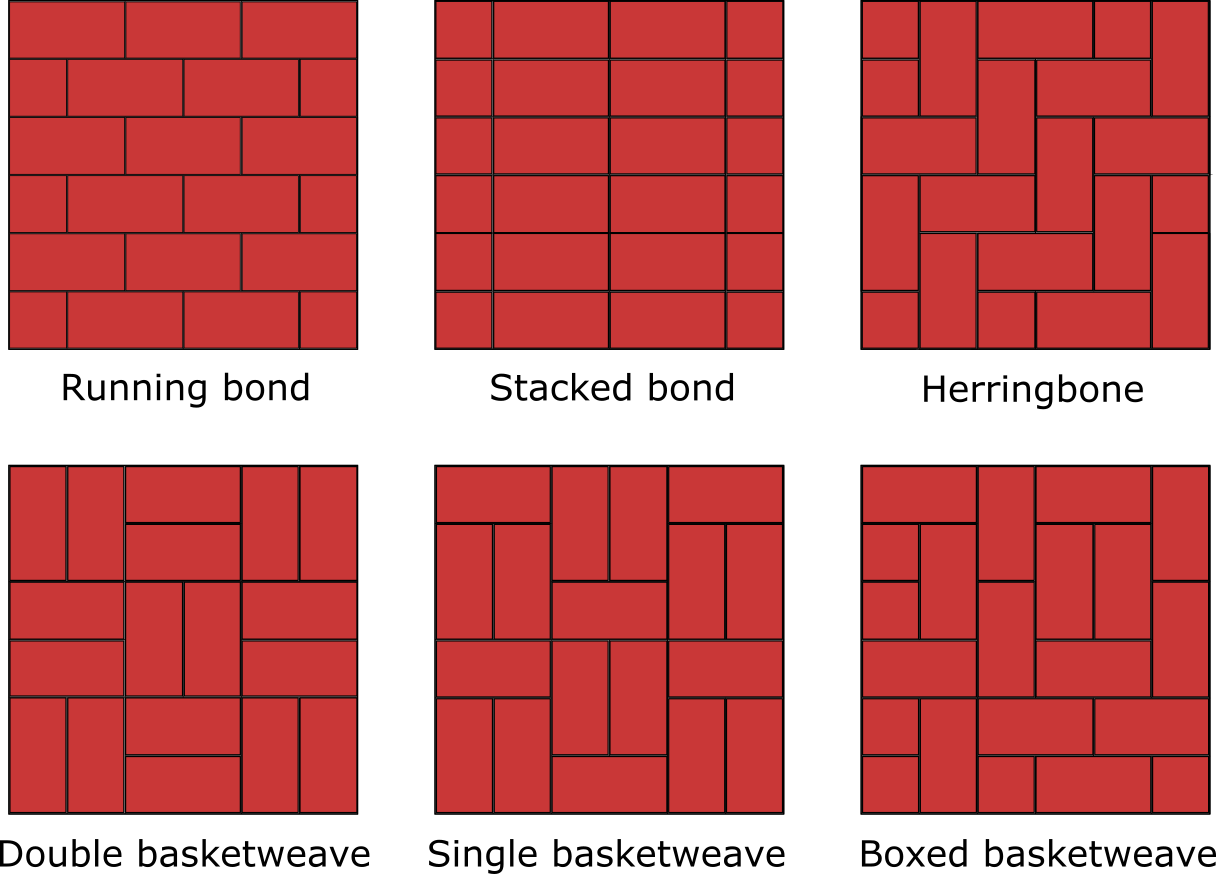# Paver Calculator

Created by Maria Kluziak
Reviewed by Bogna Szyk and Jack Bowater
Last updated: Feb 15, 2022

Make sure to use this paver calculator before you start working on your patio project! If the question "how many pavers do I need?" has ever crossed your mind, this tool is going to be of invaluable help to you. By providing the pavers cost, you will be able to calculate the total cost of your patio. The patio paver calculator will also allow you to estimate the perfect amount of materials for your project and will, as a consequence, save you money, as not a single paver will go to waste.

## How to use the paver calculator?

Using the patio paver calculator is very simple. Here's a short set of instructions:

1. Determine the area of your patio. Start by choosing whether your patio is a **single rectangle** or if it has a different shape, in which case you need to divide it into identical rectangles to make the calculation easier.

• NOTE: Don't worry if the shape of your patio is impossible to divide into rectangles of the same size. If the sizes of its subareas differ, just calculate the material in each one by choosing the single rectangle option, and then sum the results up.

• For a single rectangle, input the length and the width of the patio, and you will get its area.

• For several identical rectangles, add the number of the subareas, and the calculator will show you the total area of the patio.

2. Now you need to determine the paver brick size. Provide a single brick's width and a single brick's length to get the area it covers. In the next section of this article, you will find a table of the most common paver brick sizes.

3. With all these values ready, the calculator will show you how many bricks are needed to cover one square foot, as well the total number of patio pavers you will need for your project.

4. All thats left to do is to calculate the costs:

• To estimate the total pavers cost simply state the price of a single brick, and the paver calculator will do the work for you.

• To get the estimated cost of installation, put in the cost of installation per square foot. Typically, it costs somewhere between $8 and$20, but the prices will vary depending on your location.

• The pavers cost calculator will sum up the two values above up and show you the total estimated expenses of the project, both for the pavers cost and their installation by professionals.

## Common paver sizes

The most commonly used paver brick size is 4" x 8" (10cm x 20cm in the metric system). However, other sizes are not rare at all.

Here's a table of the most widely used paver brick sizes, along with their corresponding dimensions in the metric system:

Size (in) Size (cm) Area (ft²) Area (cm²)
4 x 8 10 x 20 0.22 200
6 x 6 15 x 15 0.25 225
6 x 9 15 x 23 0.38 245
8 x 8 20 x 20 0.44 400
12 x 12 30 x 30 1.00 900
14 x 14 36 x 36 1.36 1296
12 x 18 30 x 46 1.50 1380

If you happen to need to calculate a brick's volume, the thickness is usually 2" (5 cm). The only exceptions are the 14" x 14" and the 12" x 18" bricks. In their case, the thickness is typically 3" (7.6 cm) and 1.5" (4 cm) respectively.

## Using the paver calculator - example

Let's go through an example using the patio paver calculator together.

John decided to have his patio paved with bricks. Naturally, his first thought was, "How many pavers do I need?" His calculations went as follows:

1. John measured the total area of the patio. John's patio is a shape resembling a four-armed star, so he can divide it into five 15'x15' squares. To count this, he uses the following equation:

• patio area = subarea width * subarea length * number of subareas

• So, in our example's case:

patio area = 15 ft * 15 ft * 5 = 1,125 ft²

2. Next, John has to calculate the area a single patio paver covers and the number of pavers per square foot. He opted for square 6"x6" pavers.

• After multiplying the brick's length by its width John knows that the area of a single paver is 36 in².

• To calculate how many patio pavers fit in one square foot, the following formula is needed:

pavers per square foot = 144 / single brick's area

• The reason why the numerator is 144 is that there are 144 square inches in one square foot. In John's example:

pavers per square foot = 144 / 36 = 4

3. What's left to do is to calculate the total number of pavers needed. To do this, John uses this equation:

• total number of pavers = pavers per square foot * total project area (rounded up - if you don't get a full number, you might need to round it up and adjust the extra brick around the edges of the project)

• So the actual calculation goes like this:

total number of pavers = 4 * 1,125 = 4,500

4. John also wants to estimate the total pavers cost. He wants to buy pavers that are $0.50. • pavers cost = 4,500 *$0.50 = $2,250 • He would also like to have the pavers installed by a professional. In his area, the cost of such service is$9 per square foot, so:

installation cost = patio area * cost per ft² = 1,125 * $9 =$10,125

## A note on using the paver calculator

As is the case with all construction works, paving is prone to mistakes. To avoid having to waste gasoline going back and forth to the store for additional materials, consider buying at least 10% more of everything.

If you need help calculating the right amount of additional materials, try using our percentage calculator to remove any doubts you might have.

Patio pavers are not the only thing you need to buy for your project. Remember to take into account paver sand and gravel, which are very important materials when paving your patio.

Calculating how many pavers you need seems nice and easy when we're talking about straightforward, rectangular bricks laid out next to one another. But you may be wondering, what about patterns? How many pavers do you need then? Don't worry - the solution to this problem is quite simple as well.

The trick is to assemble one repeating part of the pattern and calculating its area, then multiplying by the times the pattern repeats.

For example:In the case of this particular pattern, to calculate the area, you would have to use the following formula:

how many sets of the pattern are needed = total project area / (a * b + c * d + e * f + g * h)

Here are some of the most popular patterns that can be created by the standard 4" x 8" paver bricks.• Running bond - mostly associated with simple brickwork, running bond is one of the most common and most natural paving patterns to create. It can also be created with square bricks.

• Stacked bond - not very commonly used with pavers, but very easy and simple to create. Might be a good idea if it's you are doing your paving on your own.

• Herringbone - the illustration shows a 90° herringbone pattern. 45° is equally popular.

• Basketweave - basketweave patterns create the illusion of long bricks disappearing under each other, just like in real baskets. There are countless variations of this kind of patio paver pattern.

## Why is having a paved patio a good idea?

There is a number of reasons why we think it's a great idea to have your patio done with pavers.

1. Paved patios are durable. Pavers are usually made of concrete, clay brick or even natural stone. There aren't many construction materials stronger than that. Pavers rarely crack, and you can expect them to remain unchanged for many years.

2. They do well in varying weather. Solid surfaces, such as concrete, are prone to cracking and generally don't fare very well in places where the temperature changes drastically between seasons. You can see it on many roads - notice how many new potholes tend to appear after winter. With pavers, this will not be an issue.

3. They are low maintenance. As stated above, pavers are incredibly durable compared to solid surfaces, so you won't have to make many repairs. Even if you do have to make some repairs, it usually suffices to remove a tiny part of the patio rather than, say, a whole slab of concrete. As for cleaning, it's often enough to use a little water with soap to make the patio look like it's brand new.

Maria Kluziak
Project area
Area shape
Single rectangle
Width
ft
Length
ft
Project area
ft²
Paver brick size
Width
in
Length
in
Brick size
in²
Pavers needed
Pavers
per
ft²
Number of pavers
The costs
Paver price
$Cost of installation$ per
ft²
Pavers cost
$Total installation cost$
Total expenses
\$
People also viewed…

### Black Hole Collision Calculator

The Black Hole Collision Calculator lets you see the effects of a black hole collision, as well as revealing some of the mysteries of black holes, come on in and enjoy!

### K-factor

Use our K-factor calculator to determine the position of the neutral axis in the sheet of metal you are planning to bend.

### Limestone

Find the volume and weight of limestone rocks you will need for your project using this easy-to-use limestone calculator.

### Podcasts

Do you feel like you could be doing something more productive or educational while on a bus? Or while cleaning the house? Well, why don't you dive into the rich world of podcasts! With this podcast calculator, we'll work out just how many great interviews or fascinating stories you can go through by reclaiming your 'dead time'!